1. /
2. CBSE
3. /
4. Class 09
5. /
6. Mathematics
7. /
8. CBSE Question Paper 2017...

# CBSE Question Paper 2017 class 12 Mathematics### myCBSEguide App

Download the app to get CBSE Sample Papers 2023-24, NCERT Solutions (Revised), Most Important Questions, Previous Year Question Bank, Mock Tests, and Detailed Notes.

CBSE Question Paper 2017 class 12 Mathematics conducted by Central Board of Secondary Education, New Delhi in the month of March 2017. CBSE previous year question papers with solution are available in myCBSEguide mobile app and cbse guide website. The Best CBSE App for students and teachers is myCBSEguide which provides complete study material and practice papers to cbse schools in India and abroad.

CBSE Question Paper 2017 class 12 Maths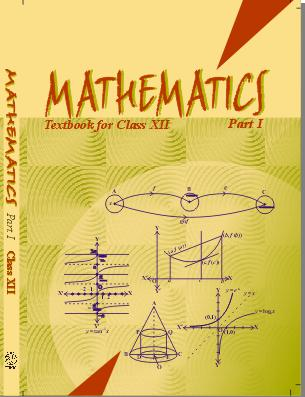## Class 12 Mathematics list of chapters

1. Relations and Functions
2. Inverse Trigonometric Functions
3. Matrices
4. Determinants
5. Continuity and Differentiability
6. Application of Derivatives
7. Integrals
8. Application of Integrals
9. Differential Equations
10. Vector Algebra
11. Three Dimensional Geometry
12. Linear Programming
13. Probability

## CBSE Last Year Paper class 12 Mathematics

### General Instructions:

1. All questions are compulsory.
2. This question paper consists of 29 questions divided into four sections A, B, C and D. Section A comprises of 4 questions of one mark each, Section B comprises of 8 questions of two marks each, Section C comprises of 11 questions of four marks each and Section D comprises of 6 questions of six marks each.
3. All questions in Section A are to be answered in one word, one sentence or as per the exact requirement of the question.
4. There is no overall choice. However, internal choice has been provided in 3 questions of four marks each and 3 questions of six marks each. You have to attempt only one of the alternatives in all such questions.
5. Use of calculators is not permitted. You may ask for logarithmic tables, if required.

### SECTION – A Question numbers 1 to 4 carry 1 mark each.

1. Let A and B are matrices of order 3 × 2 and 2 × 4 respectively. Write order of matrix (AB).
2. Write the equation of tangent drawn to the curve y = sin x at the point (0, 0).
3. Find :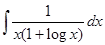4. Write the angle between the vectors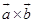and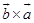.

### SECTION – B Question numbers 5 to 12 carry 2 marks each.

5. In the following matrix equation use elementary operation R2 → R2 + R1 and write the equation thus obtained.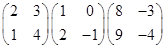6. Find the value of k for which the function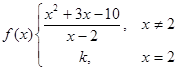is continuous at x = 2.
7. The radius r of a right circular cone is decreasing at the rate of 3 cm/minute and the height h is increasing at the rate of 2 cm/minute. When r = 9 cm and h = 6 cm, find the rate of change of its volume.
8. Find: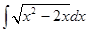9. Find the differential equation of the family of curves y2 = 4 ax.
10. Find the general solution of the differential equation11. If the points with position vectors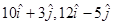and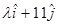are collinear, find the value of λ.
12. A firm has to transport at least 1200 packages daily using large vans which carry 200 packages each and small vans which can take 80 packages each.

The cost for engaging each large van is ` 400 and each small van is Rs.200.Not more than Rs.3,000 is to be

spent daily on the job and the number of large vans cannot exceed the number of small vans. Formulate this problem as a LPP given that the objective is to minimize cost.

### SECTION – C Question numbers 13 to 23 carry 4 marks each.

13. Prove that :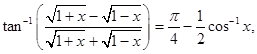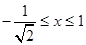14.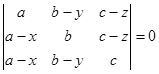then using properties of determinants, find the value of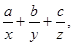where x, y, z ≠ 0.
OR Using elementary operations, find the inverse of the following matrix A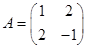.
15. If x = a (cos θ + θ sin θ) and y = a (sin θ – θ cos θ), then find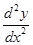.

These are 15 questions only. To view and download complete question paper with solution install myCBSEguide App from google play store or login to our student dashboard.

## CBSE Question Paper 2017 class 12 Mathematics

Download class 12 Mathematics question paper with solution from best CBSE App the myCBSEguide. CBSE class 12 Mathematics question paper 2018 in PDF format with solution will help you to understand the latest question paper pattern and marking scheme of the CBSE board examination. You will get to know the difficulty level of the question paper. CBSE question papers 2016 for class 12 Mathematics have 14 questions with solution.

## CBSE Question Paper

CBSE question papers 2018, 2017, 2016, 2015, 2014, 2013, 2012, 2011, 2010, 209, 2008, 2007, 2006, 2005 and so on for all the subjects are available under this download link. Practicing real question paper certainly helps students to get confidence and improve performance in weak areas.

To download CBSE Question Paper 2017 class 12 Accountancy, Chemistry, Physics, History, Political Science, Economics, Geography, Computer Science, Home Science, Accountancy, Business Studies and Home Science; do check myCBSEguide app or website. myCBSEguide provides sample papers with solution, test papers for chapter-wise practice, NCERT solutions, NCERT Exemplar solutions, quick revision notes for ready reference, CBSE guess papers and CBSE important question papers. Sample Paper all are made available through the best app for CBSE students and myCBSEguide website.### Test Generator

Create question paper PDF and online tests with your own name & logo in minutes.### myCBSEguide

Question Bank, Mock Tests, Exam Papers, NCERT Solutions, Sample Papers, Notes